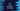# C++ different ways to find the length of a string# Introduction :

String is a sequence of characters. To find out the length of a string, we can use one loop to iterate and count the characters in a string. C++ provides a couple of other methods to find out the length without iterating. In this tutorial, I will show you how to get the length by iterating through the characters one by one and also by using the inbuilt methods.

## C++ program to find the length of a string using a loop :

The constructor of the C++ string class creates one C style string, i.e. the end element is \0. So, using a loop, we can iterate the string starting from its first element to \0 to find out the length.

The below example uses one for loop to find the length :

``````#include <iostream>
using namespace std;

int main(int argc, char const *argv[])
{
string s = "codevscolor.com";
int size = 0;

for (int i = 0; s[i] != '\0'; i++)
{
size++;
}

cout << "Size :" << size << endl;
return 0;
}``````

If you run this program, it will print 15 as the output. You can also use one while loop to find out the length in a similar way.

## C++ program to find the string length using inbuilt methods :

C++ string has two inbuilt methods to find out the length : size() and length(). We can call these methods directly on any string variable. No need to include anything.

``````#include <iostream>
using namespace std;

int main(int argc, char const *argv[])
{
string s = "codevscolor.com";

cout << "Size using size() : " << s.size() << endl;
cout << "Size using length() : " << s.length() << endl;
return 0;
}``````

It will print the below output :

``````Size using size() : 15
Size using length() : 15``````

## Using C-style string :

We can also use strlen function defined in string.h header file to find out the length of a string. The only thing required is to change the string to C style string using cstr()_ method.

``````#include <iostream>
#include <string.h>
using namespace std;

int main(int argc, char const *argv[])
{
string s = "codevscolor.com";

cout << "Size : " << strlen(s.c_str()) << endl;
return 0;
}``````

It will print the same output.